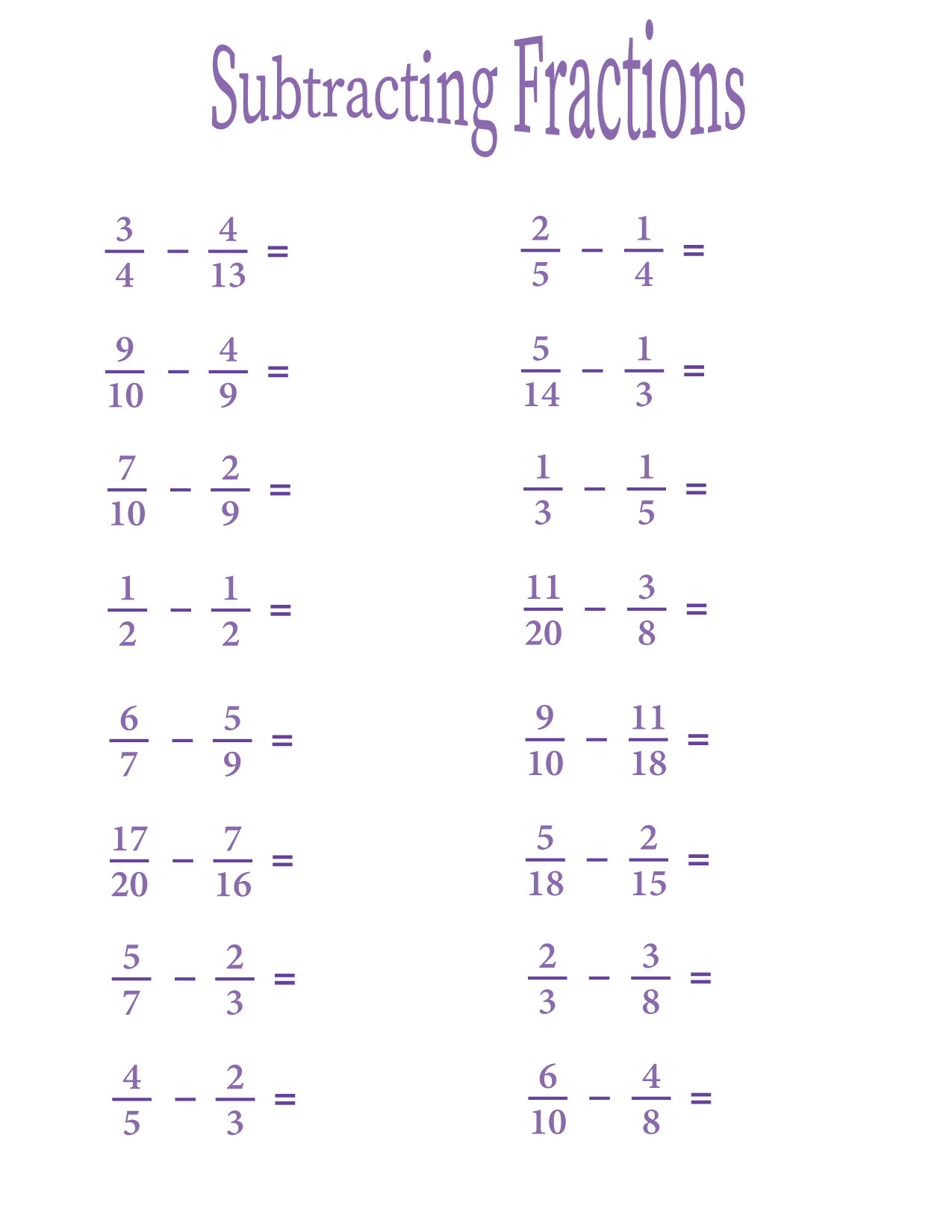After students learn what fractions are, the next step is learning how to add and subtract them. Fractions with the same (or ‘like’) denominators are easier to add and subtract because it simply involves working with the numerator.

However, it is important to learn how to add and subtract fractions with unlike denominators. This is trickier, because it means that the denominator has to be adapted in order to complete the function.

In this post, we have worksheets with adding and subtracting fractions with unlike denominators. If you finish these worksheets, you can check out other fraction worksheets here. Once students get comfortable with adding and subtracting fractions, they can start multiplying and dividing them.

In order to add fractions with unlike denominators, there is a 3-step process. The first step is to make sure the denominators are the same. This can be done by finding the least common multiple (LCM) of the denominators.

For example, if the fractions are 5/6 and 1/12, the LCM is 12, because 6 can be multiplied by 2 to create 12. In this case, you multiply both the numerator and denominator of 5/6 by 2. You would end up with 10/12, and if that is added to 1/12, the answer is 11/12.In this worksheet, some of the numerators will be larger than the denominators. It is okay to write it this way, but as students get older, teachers might want them to write mixed fractions, with the whole number as well as the fraction. This worksheet can help them practice that.## Subtracting fractions worksheet #1

The principles of adding fractions with unlike denominators also applies to subtracting them. Our next two worksheets will help you practice subtracting unlike fractions.